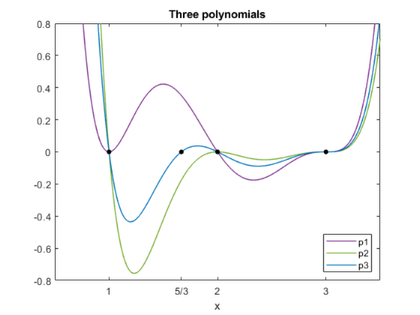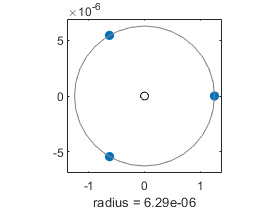# Pejorative Manifolds of Polynomials and Matrices, part 2

In an unpublished 1972 technical report "Conserving confluence curbs ill-condition," Velvel Kahan coined the descriptive term pejorative manifold. In case you don't use it in everyday conversation, pejorative means "expressing contempt or disapproval."

Velvel's report concerns polynomials with multiple roots, which are usually regarded with contempt because they are so badly conditioned. But Velvel's key observation is that although multiple roots are sensitive to arbitrary perturbation, they are insensitive to perturbations that preserve multiplicity.

Part 1 was about polynomials. This part is about matrix eigenvalues.

### Contents

#### The manifold

The pejorative manifold $\mathcal{M}$ is now the set of all 6-by-6 matrices which have an eigenvalue of multiplicity three at $\lambda$ = 3. These are severe restrictions, of course, and $\mathcal{M}$ is a tiny subset of the set of all matrices. But if we stay within $\mathcal{M}$, life is not nearly so harsh.

#### Two matrices

The Jordan Canonical Form of a matrix is bidiagonal, with eigenvalues on the diagonal and 1's and 0's on the super-diagonal. In our situation here, each eigenvalue with multiplicity m has a single m-by-m Jordan block with 1's on the superdiagonal. The Jordan blocks for distinct eigenvalues are separated by a zero on the super-diagonal.

Our first matrix has a 3-by-3 block with $\lambda$ = 3, then a 1-by-1 block with $\lambda$ = 2, and finally a 2-by-2 block with $\lambda$ = 1, so the diagonal is

   d = [3  3  3  2  1  1];


and the superdiagonal is

   j = [1  1  0  0  1];


Here it is

   J1 = diag(j,1)
A1 = diag(d) + J1

J1 =
0     1     0     0     0     0
0     0     1     0     0     0
0     0     0     0     0     0
0     0     0     0     0     0
0     0     0     0     0     1
0     0     0     0     0     0
A1 =
3     1     0     0     0     0
0     3     1     0     0     0
0     0     3     0     0     0
0     0     0     2     0     0
0     0     0     0     1     1
0     0     0     0     0     1


Our second matrix moves one super-diagonal element to swap the multiplicities of $\lambda$ = 2 and $\lambda$ = 1.

   J2 = diag([1 1 0 1 0],1)
A2 = diag([3 3 3 2 2 1]) + J2

J2 =
0     1     0     0     0     0
0     0     1     0     0     0
0     0     0     0     0     0
0     0     0     0     1     0
0     0     0     0     0     0
0     0     0     0     0     0
A2 =
3     1     0     0     0     0
0     3     1     0     0     0
0     0     3     0     0     0
0     0     0     2     1     0
0     0     0     0     2     0
0     0     0     0     0     1


These two matrices were constructed to have the two polynomials from my last post as characteristic polynomials. There is no need to compute the zeros, they are on the diagonals.

   p1 = charpoly(A1,'s');
p2 = charpoly(A2,'s');


$$p1 = s^6-13\,s^5+68\,s^4-182\,s^3+261\,s^2-189\,s+54$$

$$p2 = s^6-14\,s^5+80\,s^4-238\,s^3+387\,s^2-324\,s+108$$

#### Convex combination

The convex linear combination puts the weights on the superdiagonal and the new eigenvalue of the diagonal.

   format short
A = 1/3*A1 + 2/3*A2

A =
3.0000    1.0000         0         0         0         0
0    3.0000    1.0000         0         0         0
0         0    3.0000         0         0         0
0         0         0    2.0000    0.6667         0
0         0         0         0    1.6667    0.3333
0         0         0         0         0    1.0000


#### Characteristic polynomial

Let's check that the characteristic polynomial is the same as the third polynomial we had last time.

   p3 = charpoly(A,'s');


$$p3 = s^6-\frac{41\,s^5}{3}+76\,s^4-\frac{658\,s^3}{3}+345\,s^2-279\,s+90$$

#### Plot

The plots of the three polynomials show how the triple root is more sensitive than either double root, which are, in turn, more sensitive than any of the simple roots.

   plot_polys(p1,p2,p3)#### Similarity transformation

A similarity transformation preserves, but disguises, the eigenvalues. Because it's handy, I'll use the HPL-AI matrix from my blog post last December HPL-AI Benchmark.

   format short
M = HPLAI(6,-1)

M =
1.1667    0.1429    0.1250    0.1111    0.1000    0.0909
0.2000    1.1667    0.1429    0.1250    0.1111    0.1000
0.2500    0.2000    1.1667    0.1429    0.1250    0.1111
0.3333    0.2500    0.2000    1.1667    0.1429    0.1250
0.5000    0.3333    0.2500    0.2000    1.1667    0.1429
1.0000    0.5000    0.3333    0.2500    0.2000    1.1667


#### Yes ma'am

Here's how we do a similarity transformation in MATLAB.

   B = M*A/M

B =
3.0610    1.1351    0.0899   -0.1695   -0.1178   -0.2053
0.0405    3.1519    1.1018   -0.1919   -0.1296   -0.2244
0.1360    0.2922    3.2144   -0.1402   -0.0745   -0.1867
0.1096    0.3808    0.2786    1.8527    0.6013   -0.1919
0.2839    0.6709    0.4447   -0.1222    1.6349    0.1467
1.5590    1.5424    0.7469   -0.1300   -0.0449    0.7517


#### Eigenvalues

What did this do to the eigenvalues?

   format long
e = eig(B)

e =
1.000000000000000 + 0.000000000000000i
1.666666666666671 + 0.000000000000000i
1.999999999999999 + 0.000000000000000i
3.000006294572211 + 0.000000000000000i
2.999996852713897 + 0.000005451273553i
2.999996852713897 - 0.000005451273553i


The simple roots have hardly moved. The root with multiplicity three is perturbed by roughly the cube root of precision.

   format short
e3 = e(4:6) - 3;
r3 = abs(e3)
circle(e3)

r3 =
1.0e-05 *
0.6295
0.6295
0.6295#### Eigenvectors

What about the eigenvectors?

   [V,~] = eig(B);
imagV = imag(V)
realV = real(V)

imagV =
1.0e-05 *
0         0         0         0         0         0
0         0         0         0    0.3705   -0.3705
0         0         0         0    0.0549   -0.0549
0         0         0         0    0.0678   -0.0678
0         0         0         0    0.0883   -0.0883
0         0         0         0    0.1225   -0.1225
realV =
-0.0601   -0.0519   -0.0904   -0.6942    0.6942    0.6942
-0.0664   -0.0590   -0.1017   -0.1190    0.1190    0.1190
-0.0742   -0.0683   -0.1162   -0.1487    0.1487    0.1487
-0.3413   -0.9310   -0.9488   -0.1983    0.1983    0.1983
0.2883    0.3258   -0.1627   -0.2975    0.2975    0.2975
-0.8870   -0.1275   -0.2033   -0.5950    0.5950    0.5950


The last two vectors have small imaginary components and their real components are nearly the same as the fourth vector. So only the first four columns of V are good eigenvectors. We see B, and hence A, is defective. It does not have a full set of linearly independent eigenvectors.

#### Condition of eigenvalues

   help condeig
format short e
kappa = condeig(B)

 CONDEIG Condition number with respect to eigenvalues.
CONDEIG(A) is a vector of condition numbers for the eigenvalues
of A. These condition numbers are the reciprocals of the cosines
of the angles between the left and right eigenvectors.
[V,D,s] = CONDEIG(A) is equivalent to:
[V,D] = EIG(A); s = CONDEIG(A);

Large condition numbers imply that A is near a matrix with
multiple eigenvalues.

Class support for input A:
float: double, single

Documentation for condeig
doc condeig

kappa =
1.6014e+00
2.9058e+00
2.9286e+00
1.3213e+10
1.3213e+10
1.3213e+10


Look carefully at those numbers -- the power of 10 on the first three is zero, while on the last three it is 10. This confirms that the first three eigenvalues are well conditioned, while the fourth is not.

#### Jordan Canonical Form

A is almost its own JCF, so this is no surprise.

   A_jcf = jordan(A)

A_jcf =
3.0000e+00   1.0000e+00            0            0            0            0
0   3.0000e+00   1.0000e+00            0            0            0
0            0   3.0000e+00            0            0            0
0            0            0   1.0000e+00            0            0
0            0            0            0   1.6667e+00            0
0            0            0            0            0   2.0000e+00


But what about B? With exact computation, it would have the same JCF.

   format short e
B_jcf = jordan(B);
real_B_jcf = real(B_jcf)
imag_B_jcf = imag(B_jcf)

real_B_jcf =
1.0000e+00            0            0            0            0            0
0   1.6667e+00            0            0            0            0
0            0   2.0000e+00            0            0            0
0            0            0   3.0000e+00            0            0
0            0            0            0   3.0000e+00            0
0            0            0            0            0   3.0000e+00
imag_B_jcf =
0            0            0            0            0            0
0            0            0            0            0            0
0            0            0            0            0            0
0            0            0            0            0            0
0            0            0            0  -2.7873e-06            0
0            0            0            0            0   2.7873e-06


The computed JCF is diagonal. This is, yet again, an example of the fact that the The Jordan Canonical Form Just Does't Compute. Also see Golub and Wilkinson.

#### And The Beat Goes On ...

I was just about to make that the end of the post when I tried this. I didn't realize it at the time, but the use of the HPL-AI matrix for the "random" similarity transformation has some welcome consequences. The elements of this matrix are the ratios of small integers.

   M = sym(M)

M =
[ 7/6, 1/7, 1/8, 1/9, 1/10, 1/11]
[ 1/5, 7/6, 1/7, 1/8,  1/9, 1/10]
[ 1/4, 1/5, 7/6, 1/7,  1/8,  1/9]
[ 1/3, 1/4, 1/5, 7/6,  1/7,  1/8]
[ 1/2, 1/3, 1/4, 1/5,  7/6,  1/7]
[   1, 1/2, 1/3, 1/4,  1/5,  7/6]


The elements of A are also the ratios of small integers.

   sym(A)

ans =
[ 3, 1, 0, 0,   0,   0]
[ 0, 3, 1, 0,   0,   0]
[ 0, 0, 3, 0,   0,   0]
[ 0, 0, 0, 2, 2/3,   0]
[ 0, 0, 0, 0, 5/3, 1/3]
[ 0, 0, 0, 0,   0,   1]


The elements of inv(M) are ratios of large integers, but I don't have to show them because we're not going to invert M, even symbolically. Instead we use forward slash to compute the similarity transformation.

   B = M*A/M;


The elements of B are also the ratios of large integers. Let's just look at the first column; the other columns are similar.

    B1 = B(:,1)

B1 =
5261534240243927141/1718872313588352715
69679116377352174/1718872313588352715
46738873967260860/343774462717670543
2898457606578534/26444189439820811
97602262779214116/343774462717670543
2679724812276211392/1718872313588352715


#### JCF

With this exact symbolic computation, the characteristic polynomial of B is p3.

   charpoly(B,'s')

ans =
s^6 - (41*s^5)/3 + 76*s^4 - (658*s^3)/3 + 345*s^2 - 279*s + 90


As a result, the JCF of B is correct.

   jordan(B)

ans =
[ 1,   0, 0, 0, 0, 0]
[ 0, 5/3, 0, 0, 0, 0]
[ 0,   0, 2, 0, 0, 0]
[ 0,   0, 0, 3, 1, 0]
[ 0,   0, 0, 0, 3, 1]
[ 0,   0, 0, 0, 0, 3]


#### Symbolic Eigenvectors

The third output argument of the symbolic version of eig is a vector whose length is the number of linearly independent eigenvectors. This is the sum of the geometric multiplicities. In this case, it is 4.

   [V,E,k] = eig(B)

V =
[  11/27,  463/6831,  4/9, 7/6]
[  25/54,    31/414,  1/2, 1/5]
[  15/28,  485/5796,  4/7, 1/4]
[ 460/63, 1115/2898, 14/3, 1/3]
[  -23/9,  -157/483,  4/5, 1/2]
[      1,         1,    1,   1]
E =
[ 5/3, 0, 0, 0, 0, 0]
[   0, 1, 0, 0, 0, 0]
[   0, 0, 2, 0, 0, 0]
[   0, 0, 0, 3, 0, 0]
[   0, 0, 0, 0, 3, 0]
[   0, 0, 0, 0, 0, 3]
k =
1     2     3     4


#### Check

Verify that the eigenvectors work.

   BV = B*V

VE_k = V*E(k,k)

BV =
[    55/81,  463/6831,  8/9, 7/2]
[  125/162,    31/414,    1, 3/5]
[    25/28,  485/5796,  8/7, 3/4]
[ 2300/189, 1115/2898, 28/3,   1]
[  -115/27,  -157/483,  8/5, 3/2]
[      5/3,         1,    2,   3]
VE_k =
[    55/81,  463/6831,  8/9, 7/2]
[  125/162,    31/414,    1, 3/5]
[    25/28,  485/5796,  8/7, 3/4]
[ 2300/189, 1115/2898, 28/3,   1]
[  -115/27,  -157/483,  8/5, 3/2]
[      5/3,         1,    2,   3]


#### TKP Preview

While preparing these two posts about "pejorative manifolds", I discovered some beautiful patterns of roundoff error generated by Triple Kronecker Products with very high multiplicity. That is going to be my next post. Here is a preview. tkp_preview.gif

   close


Published with MATLAB® R2019b

|